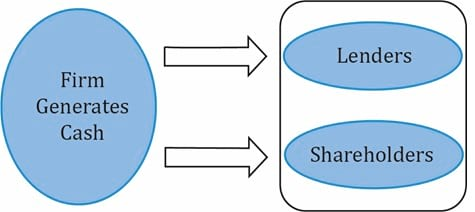Understanding Cash Flow Statements | IFT World
IFT Notes for Level I CFA® Program
IFT Notes for Level I CFA® Program

# Part 4

## 16. Cash Flow Statement Analysis: Evaluation of Sources and Uses of Cash

Evaluation of the cash flow statement should involve the following:

• Evaluate where the major sources and uses of cash flow are between operating, investing and financing activities. Major sources of cash for a company can vary with its stage of growth. For example, for a mature company it is expected that operating activities are the primary source of cash flows. However, for all companies analysts must analyze whether operating cash flows are positive and cover capital expenditures.
• Evaluate the primary determinants of operating cash flow. Analysts should compare operating cash flow with net income. If a company has large net income but poor operating cash flow, it may be a sign of poor earnings quality. In addition, analysts need to look at consistency of operating cash flows.
• Evaluate the primary determinants of investing cash flow. This is useful for letting the analyst know how much is being invested for the future in property, plant and equipment and how much is put aside in liquid investments.

## 17. Cash Flow Statement Analysis: Common-Size Analysis

In common-size analysis of a company’s cash flow statement, there are two alternative approaches. In the first approach, we express each line item of cash inflow (outflow) as a percentage of total inflows (outflows). An example of a common-size cash flow statement using this approach is shown below for K2 Corp.

 Inflows Actual % of Total Inflow Net cash provided by operating activities 3,200 80 % Sale of Equipment 800 20% Total 4,000 100% Outflows Actual % of Total Outflow Purchase of equipment 1,500 36.58% Retirement of long-term debt 500 12.19% Retirement of common stock 325 7.9% Dividend payments 1,775 43.29% Total 4,100 100% Net increase (decrease) in cash (100)

In the second approach, we express each line item as a percentage of revenue. An example of such a statement is shown below for K2 Corp. In this example, we have assumed total revenue is 10,000.

 Cash flow Actual % of Total Revenue Cash flow from operating activities Net Income 4,000 40% Depreciation expense 500 5% Increase in accounts receivable (500) (5%) Increase in inventory (1,000) (10%) Decrease in prepaid expenses 1,000 10% Increases in accounts payable 500 5% Increases in accrued liabilities 500 5% Net cash provided by operating activities 5,000 50% Cash flow from investing activities Cash received from sale of equipment 2,000 20% Cash paid for purchase of equipment (5,000) 50% Net cash used for investing activities (3,000) (30%) Cash flow from financing activities Sale of bonds 1,000 10% Cash dividends (2,000) (20%) Net cash used for financing activities (1,000) (10%) Net increase in cash 1,000 10%

The common-size cash flow statement makes it easier to see trends in cash flow rather than just looking at the total amount. The second approach is useful for the analyst in forecasting future cash flows.

## 18. Cash Flow Analysis: Free Cash Flow to Firm and Free Cash Flow to Equity

Free cash flow to firm (FCFF) is the cash flow available to all the suppliers of capital to a company after all operating expenses have been paid and necessary investments in working capital and fixed capital have been made. The suppliers of capital include both lenders (debt) and equity shareholders (equity). This is illustrated in the figure below:The formula for computing FCFF is:

where:

FCFF = NI + NCC + Int (1- tax rate) – FCInv – WCInv

NI = Net Income

NCC = non-cash charges

Int = Interest expense

FCInv = Fixed capital investment/expenditures

WCInv = working capital expenditures

While FCFF indicates how much cash is available to all suppliers of capital, free cash flow to equity (FCFE) is the cash flow available to the company’s stockholders after all operating expenses and borrowing costs (principal and interest) have been paid and necessary investments in working capital and fixed capital have been made. The formula for computing FCFE is as follows:

FCFE = CFO – FCInv + Net Borrowing

## 19. Cash Flow Statement Analysis: Cash Flow Ratios

There are several ratios useful for the analysis of the cash flow statement. These ratios generally fall into cash flow performance (profitability) ratios and cash flow coverage (solvency) ratios. The calculation and interpretation of these ratios are summarized in the tables below.

 Performance Ratios Calculation What It Measures Cash flow to revenue CFO ÷ Net revenue Operating cash generated per dollar of revenue. Cash return on assets CFO ÷ Average total assets Operating cash generated per dollar of asset investment. Cash return on equity CFO ÷ Average shareholders’ equity Operating cash generated per dollar of owner investment. Cash to income CFO ÷ Operating income Cash generating ability of operations. Cash flow per share (CFO – Preferred dividends) ÷ Number of common shares outstanding Operating cash flow on a per-share basis.
 Coverage Ratios Calculation What It Measures Debt coverage CFO ÷ Total debt Financial risk and financial leverage. Interest coverage (CFO + Interest paid + Taxes paid) ÷ Interest paid Ability to meet interest obligations. Reinvestment CFO ÷ Cash paid for long-term assets Ability to acquire assets with operating cash flows. Debt payment CFO ÷ Cash paid for long-term debt repayment Ability to pay debts with operating cash flows. Dividend payment CFO ÷ Dividends paid Ability to pay dividends with operating cash flows. Investing and financing CFO ÷ Cash outflows for investing and financing activities Ability to acquire assets, pay debts, and make distributions to owners.